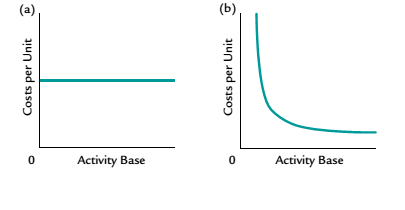Chapter 11, Problem 8CDQ

Chapter
Section
Textbook Problem

Which of the following graphs best illustrates fixed costs per unit as the activity base changes?To determine

Concept Introduction:

Variable, Fixed and Mixed Cost:

There are three types of costs according to the unit of production; Variable, Fixed and Mixed. Variable costs change proportionally with the number of units produced and variable cost per unit remains constant. Fixed Cost remains same in totality irrespective of the number of units produced. The mixed cost is the mix of variable and fixed cost, some of its part is fixed and some variable.

To Indicate:

The behavior of fixed costs per unit with change in total units produced

Explanation

There are three types of costs according to the unit of production; Variable, Fixed and Mixed. Variable costs change proportionally with the number of units produced and variable cost per unit remains constant. Fixed Cost remains same in totality irrespective of the number of units produced...

Still sussing out bartleby?

Check out a sample textbook solution.

See a sample solution

The Solution to Your Study Problems

Bartleby provides explanations to thousands of textbook problems written by our experts, many with advanced degrees!

Get Started